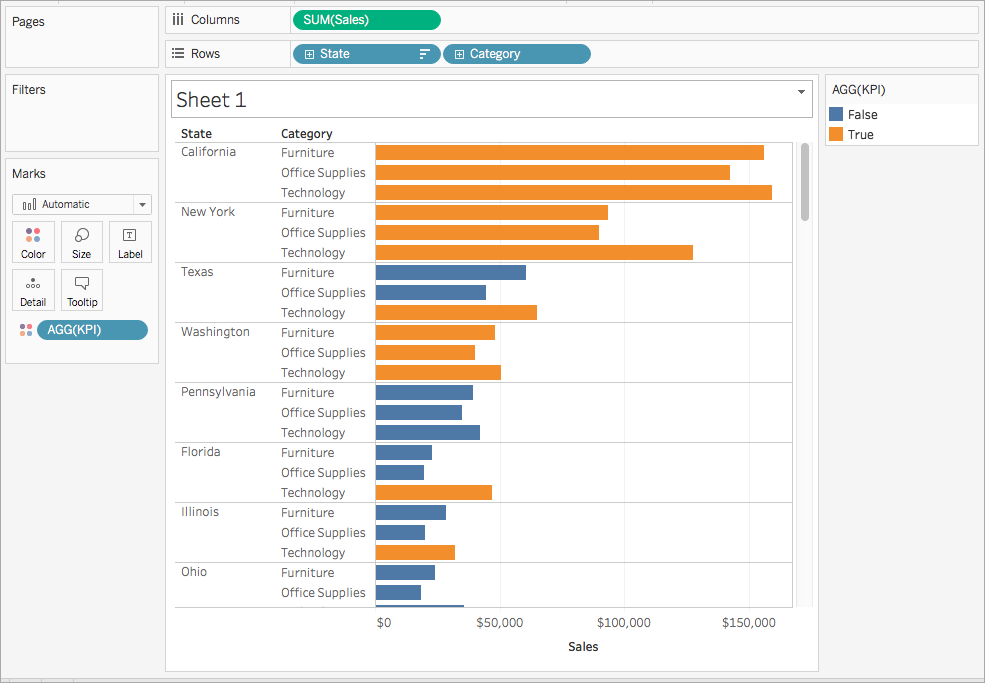## 为什么使用逻辑计算

`SUM(Sales) > 1,000,000 `

## Tableau 中的可用逻辑函数：

#### 说明

IN `<expr1> IN <expr2> `

`<expr1>` 中的值可以是集、文本值列表或合并字段。

`SUM([Cost]) IN (1000, 15, 200)`

`[SET] IN [COMBINED FIELD]`

AND

`IF <expr1> AND <expr2> THEN <then> END`

`IF (ATTR([Market]) = "Africa" AND SUM([Sales]) > [Emerging Threshold] )THEN "Well Performing"`

CASE ```CASE <expression> WHEN <value1> THEN <return1> WHEN <value2> THEN <return2> ... ELSE <default return> END```

CASE 比 IIF 或 IF THEN ELSE 更易于使用。

`CASE [Region] WHEN 'West' THEN 1 WHEN 'East' THEN 2 ELSE 3 END`

`CASE LEFT(DATENAME('weekday',[Order Date]),3) WHEN 'Sun' THEN 0 WHEN 'Mon' THEN 1 WHEN 'Tue' THEN 2 WHEN 'Wed' THEN 3 WHEN 'Thu' THEN 4 WHEN 'Fri' THEN 5 WHEN 'Sat' THEN 6 END`

ELSE `IF <expr> THEN <then> ELSE <else> END`

`If [Profit] > 0 THEN 'Profitable' ELSE 'Loss' END`

ELSEIF `IF <expr> THEN <then> [ELSEIF <expr2> THEN <then2>...] [ELSE <else>] END`

`IF [Profit] > 0 THEN 'Profitable' ELSEIF [Profit] = 0 THEN 'Breakeven' ELSE 'Loss' END`

END `IF <expr> THEN <then> [ELSEIF <expr2> THEN <then2>...] [ELSE <else>] END`

`IF [Profit] > 0 THEN 'Profitable' ELSEIF [Profit] = 0 THEN 'Breakeven' ELSE 'Loss' END`

IF `IF <expr> THEN <then> [ELSEIF <expr2> THEN <then2>...] [ELSE <else>] END`

`IF [Profit] > 0 THEN 'Profitable' ELSEIF [Profit] = 0 THEN 'Breakeven' ELSE 'Loss' END`

IFNULL `IFNULL(expr1, expr2)`

`IFNULL([Profit], 0)`

IIF `IIF(test, then, else, [unknown])`

`IIF([Profit] > 0, 'Profit', 'Loss')`

ISDATE `ISDATE(string)`

`ISDATE("2004-04-15") = True`

ISNULL `ISNULL(expression)`

`ISNULL([Profit])`

MAX `MAX(expression)``Max(expr1, expr2)`

`MAX([Sales])`

MIN `MIN(expression)``MIN(expr1, expr2)`

`MIN([Profit])`

NOT `IF NOT <expr> THEN <then> END`

`IF NOT [Profit] > 0 THEN "Unprofitable" END`

`IF [Profit] < 0 OR [Profit] = 0 THEN "Needs Improvement" END`

THEN `IF <expre> THEN <then> [ELSEIF ,expr2> THEN <then2>...] [ELSE <else>] END`

`IF [Profit] > 0 THEN 'Profitable' ELSEIF [Profit] = 0 THEN 'Break even' ELSE 'unprofitable' END`

WHEN `CASE <expr> WHEN <Value1> THEN <return1> ... [ELSE <else>] END`

`CASE [RomanNumberal] WHEN 'I' THEN 1 WHEN 'II' THEN 2 ELSE 3 END`

ZN `ZN(expression)`

`ZN([Profit])`

## 创建逻辑计算

1. 在 Tableau Desktop 中，连接到 Tableau 附带的“Sample - Superstore”已保存数据源。

2. 导航到工作表。

3. 从“数据”窗格中，将“State”（州/省/市/自治区）拖到“行”功能区。

4. 从“数据”窗格中，将“Category”（类别）拖到“行”功能区，并将其放在“State”（州/省/市/自治区）的右侧。

5. 从“数据”窗格中，将“Sales”（销售额）拖到“列”功能区。

6. 选择“分析”>“创建计算字段”

7. 在打开的计算编辑器中，执行以下操作：

• 将计算字段命名为“KPI”。

• 输入以下公式：

`SUM([Profit]) > 0`

此计算将快速检查某个成员是否大于零。如果大于零，则返回 true；否则返回 false。

• 完成后，单击“确定”

新计算字段会显示在“数据”窗格中的“度量”下。就像其他字段一样，您可以在一个或多个可视化项中使用该字段。

8. 从“数据”窗格中，将KPI拖到“标记”卡上的“颜色”

您现在可以看到每个州/省/市/自治区的哪些类别在亏损。